# How to Get the Current Sheet Number of a Workbook?

Sometimes the user is working with multiple worksheets at the same time. While working with a large number of sheets, obtaining current sheet numbers is important to track the current working operations status. To access the worksheet number, for this tutorial, we are using two strategies, the first strategy involves setting the sheet number on the status bar, and the second strategy involves using the VBA code. VBA code is an effective way to write code once, and the same code can be used to process data any number of times, by simply calling the associated module.

A list of solutions that allow the user to evaluate the current sheet number is given below:

• The first way is to display the sheet number in the status bar by enabling the option feature.

• Another way is to use the excel visual basic analysis code.

## Examples to Obtain the Current Workbook Sheet Number in Excel

### Example 1

Step 1

Consider the below-given example to enable the status bar option:

To solve the required task, consider the below given workbook: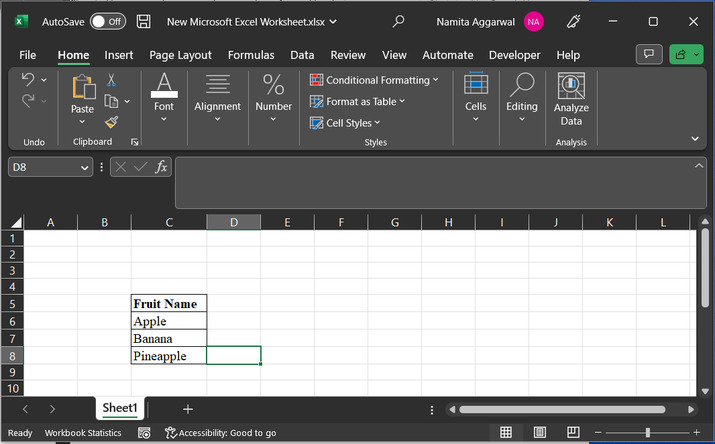Step 2

After that click on the “+” sign to add more sheets. Create 3 sheets, by clicking on “+” sign two or more times.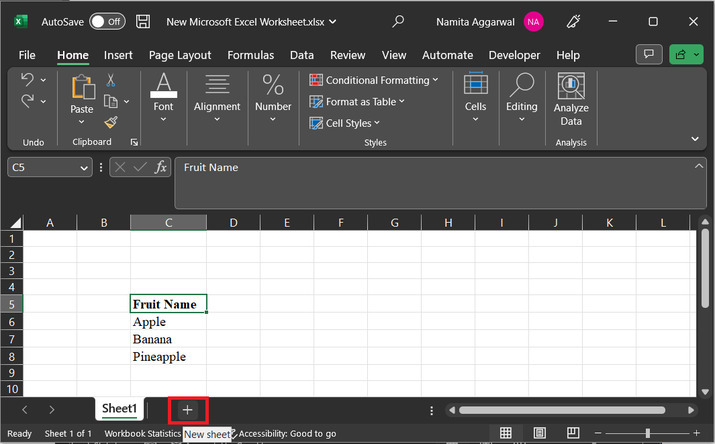Step 3

Sheets will appear at the bottom of the page: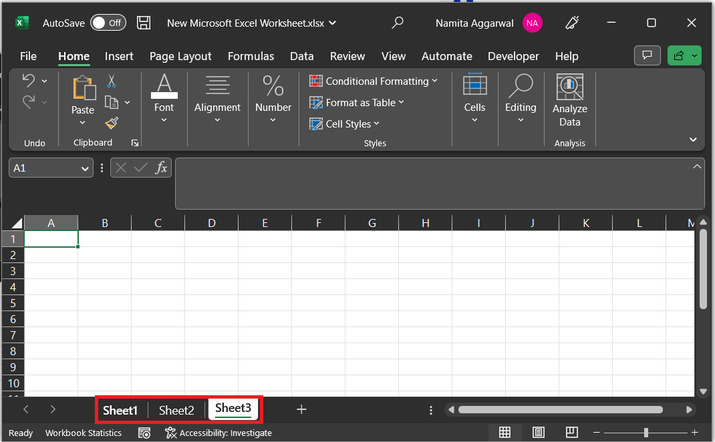Step 4

Go to the status bar of the excel sheet and right click on the sheet. Consider the area depicted below: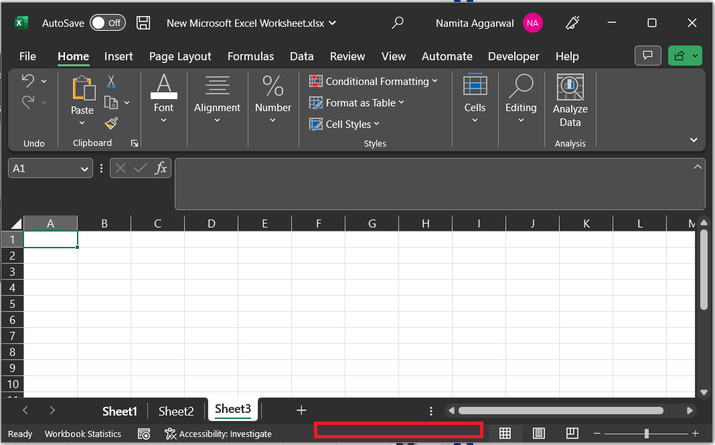Step 5

Right click will display the below given option in front of user.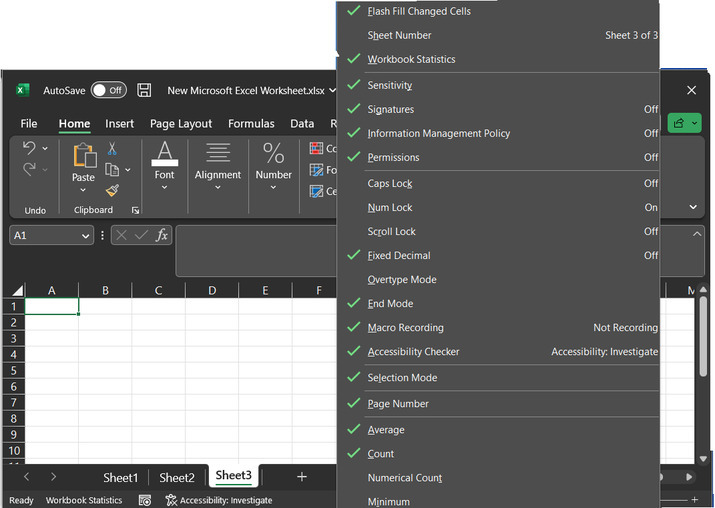Step 6

Choose the “sheet number” option.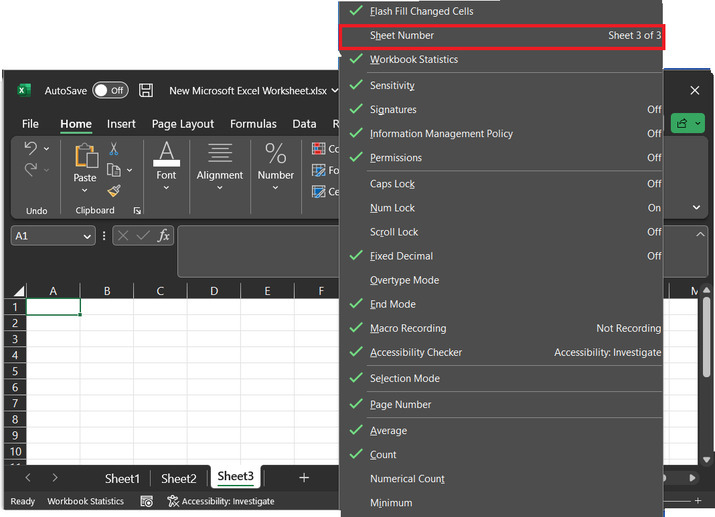Step 7

This will display the sheet number on the bottom of the excel sheet.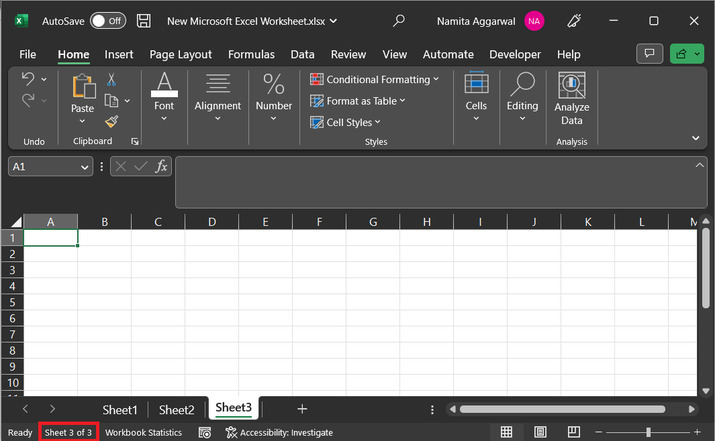This example will allow user to add the feature of current sheet number at the bottom of the excel sheet. After enabling the feature user can easily track the sheet number from the status bar.

### Example 2

Let’s consider another example to obtain the current sheet number. The below given example will demonstrate the user of VBA code to access the current sheet number.

Consider below given steps to understand the procedure:

Step 1

To solve the task by using the VBA. Firstly, go to the “Developer” tab and click on “Visual Basic” under the “code” section.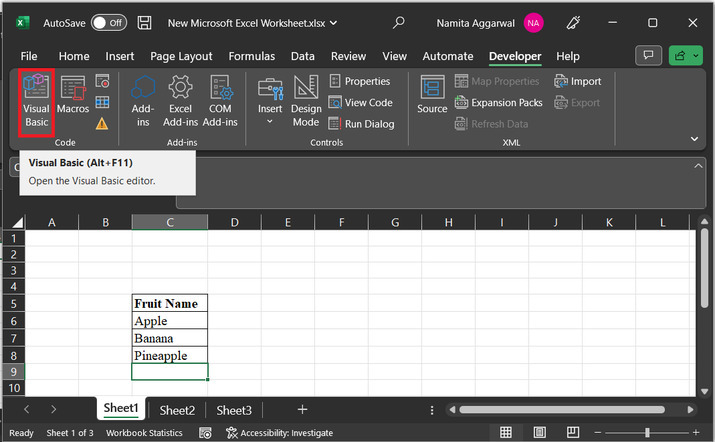Step 2

This will display the below given Microsoft VBA area: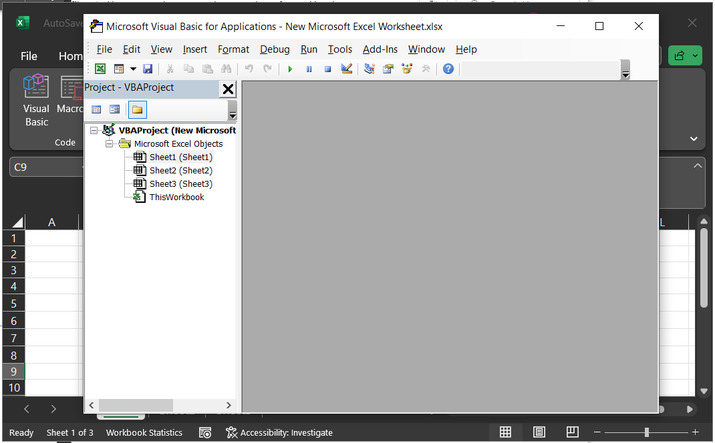Step 3

After that user need to write a module definition. This module contains code to perform the required task. To do so, click on “Insert” tab module. This will display code area to user.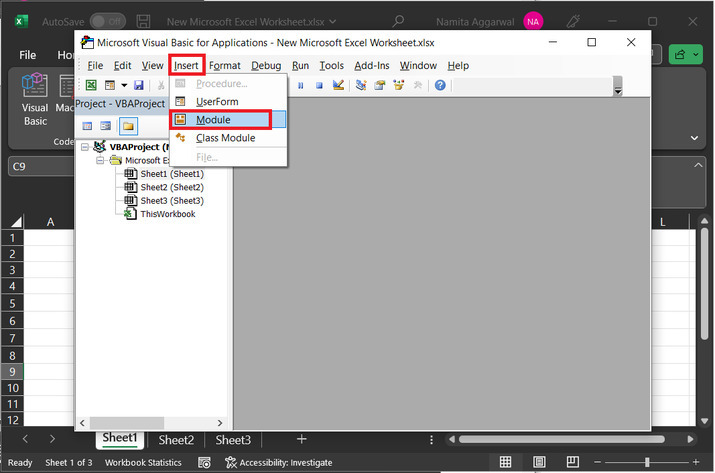Step 4

In the appeared code editor, copy the below given code:

' define module header
Sub Get_Sheet_number()
' use index instance to access sheet number
MsgBox ActiveSheet.Index
' end of defined module
End Sub


### Snapshot of code area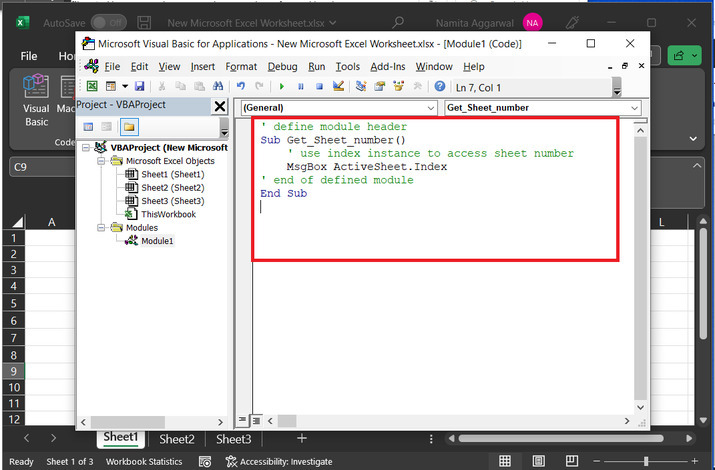Step 5

After typing the code to the code area. Now, it is the time to execute the code. To do so, click on “run” option. This will execute the provided code: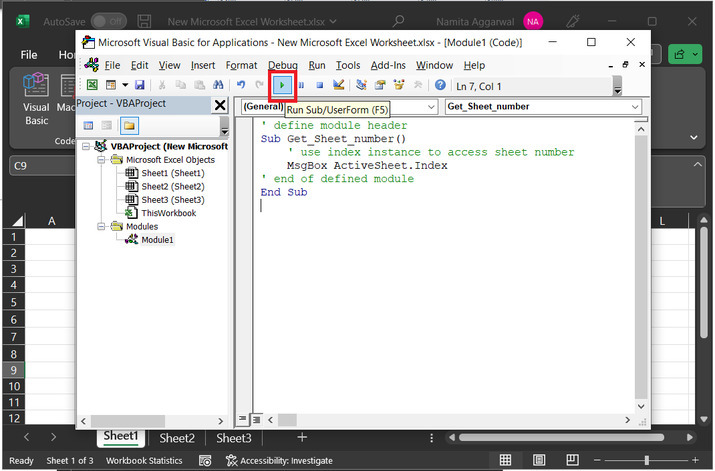Step 6

The finally obtained results are given below: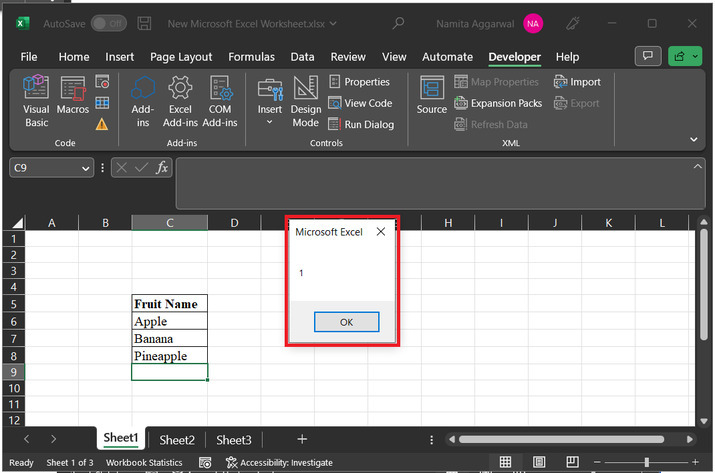## Conclusion

This tutorial illustrates two simple strategies to obtain the current sheet number. First case involved the use status bar while the other option allow user to use the VBA code. Both the process is easy to use and can process data effectively, without any issue.

Updated on: 22-Aug-2023

688 Views